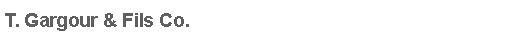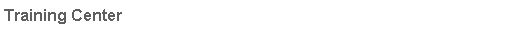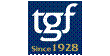Electrics & Electronics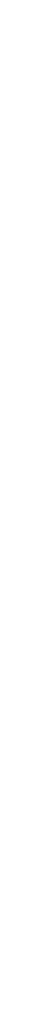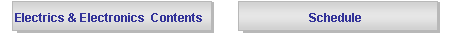Subject : Basics of Logic Circuits
 Code : EE103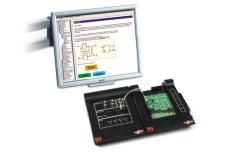Participants : Vehicle Technicians, Students from Technical Schools & Collages Contents : · Introduction to basic logic circuits  · Introduction to truth tables and symbols, logic equations and timing diagrams for each of the basic gates  · Experimental derivation of Boolean functions and laws  · Design of basic logic circuits using NAND gates and NOR-gates  · Minimisation of logic circuits with the aid of Karnaugh maps with experimental testing  · Introduction to the principle of a flip-flop  · Investigating the operation of a JK flip flop by measurement (static and dynamic input signal/single-pulse operation)  · Investigation of a counter circuit Duration: 3 Days. Prerequisites: “Basics of Electronics”, training course